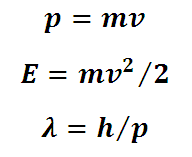# Momentum, Kinetic Energy and de-Broglie wavelength Calculator

This is a CalcTown calculator to calculate momentum, energy and de-Broglie wavelength of an electron.

#### Result

kg-m/s
Joule
meV
nmwhere

m=9.10938291 × 10-31 kg (Rest mass of the electron has been used assuming velocity of the electron to be much smaller in                                             comparison to the speed of light

h=6.62606957 × 10-34 m2 kg / s